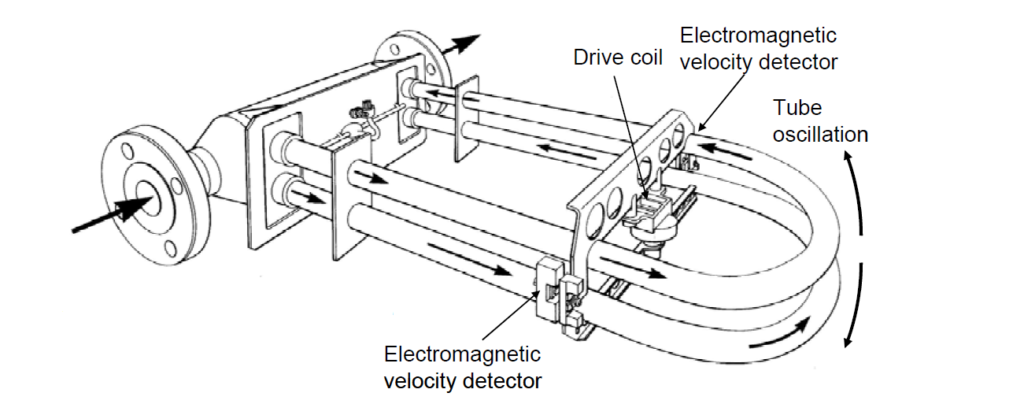# Different types mass flowmetersMass flowmeters are devices that measure the amount fluid passed to a process. Mass flowmeters are used where the composition of materials used are important, which ensures the quality of the product.

These are the different types of mass flowmeters:

### 1. Coriolis Mass Flow Meters

In this type, two parallel measuring tubes containing flowing fluid were oscillated in antiphase, acting as a tuning fork using strong electromagnets. The Coriolis forces produced in the measuring tubes cause a phase change in the oscillations of the tube.

The tubes stay standstill when there is no flow. (1).The mass flow causes the deceleration of the oscillation at the entrance of the pipes (2) and the acceleration at the exit (3). The phase difference (A-B) increases with increasing mass flow.

### 2. Thermal Mass Flowmeters

In thermal mass flowmeter method, a heated object is placed at the middle of the flow channel. The fluid convect heat from the wire, The reduction of the temperature by that heated object is a function of the true mass flow rate because the mechanism of heat loss is the speed at which the fluid molecules come into contact with the heated object, and each of those molecules has a mass definite.

### 3. Impeller-Turbine Mass Flow Meters

In this device, there are two rotating elements impeller and turbine. The impeller is driven by a synchronous motor at constant speed, through a magnetic coupling and imparts an angular velocity to the fluid as it flows through the meter.

The turbine located downstream of the impeller eliminates all the angular momentum of the fluid and, therefore, receives a torque proportional to the angular momentum. This turbine is constrained by a spring that deflects through an angle that is proportional to the torque that the fluid exerts on it, which gives a measure of mass flow.

### 4. Twin-Turbine Mass Flow Meter:

In this instrument two turbines are mounted on a common shaft. A reluctance type transducer is mounted over each turbine and a strong magnet is located in each turbine within the twin-turbine assembly.

Each turbine is designed with a different blade angle; therefore there is a tendency for the turbines
to turn at different angular velocities. When the flow happens the both turbine rotate in different angular velocity, which is the function mass flow.

### Gyroscopic Mass Flow Meter:

It consists of a tube with a circle or square shape. A motor introduces an oscillating vibration at a constant angular velocity “ω” on the axis A.

When the fluid passes through the loop, a moment of precession type occurs on the B axis and is measured by the deviation of the sensor element. It can be shown that this deviation is directly proportional to the mass flow.